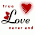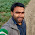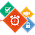# Program to Separate The Digits in an Integer Using Java

The program below is the answer to Deitel's Java How to Program (9th Edition) Chapter 2 Exercise 2.30.

Write an application that inputs one number consisting of five digits from the user, separates the number into its individual digits and prints the digits separated from one another by three spaces each. For example, if the user types in the number 42339, the program should print 4   2   3   3   9

```/**
*
* @author AGHATISE OSAZUWA
* Website: www.cscprogrammingtutorials.com
*/

import java.util.Scanner;

public class SeparatingTheDigitsInAnInteger {
public static void main (String [] args) {

Scanner value = new Scanner (System.in);

int number; //number entered by the user
int digit1; //first digit of number
int digit2; //second digit of number
int digit3; //third digit of number
int digit4; //fourth digit of number
int digit5; //fifth digit of number

System.out.println (); //displays a blank line

System.out.print ("Enter An Integer Number Consisting of Five Digits "
+ "e.g 12345: ");
number = value.nextInt();

digit5 = number % 10;
digit4 = (number % 100)/10;
digit3 = (number % 1000)/100;
digit2 = (number % 10000)/1000;
digit1 = number / 10000;

System.out.println ();

System.out.printf ("%d   %d   %d   %d   %d\n", digit1, digit2, digit3,
digit4, digit5);

}
}
```

Here is the same program to separate digits of a number written in C using another algorithm/method.

1.First of all excuse my poor english.
This program don't work properly, i think you should use:
digit5=number%10;
digit4=(number%100)/10;
digit3=(number%1000)/100;
digit2=(number%10000)/1000;
digit1=number/10000;

1.Did you run the program? Because the code works fine. I just tried it. How come yours didn't work properly? What error are you getting?

P.S. Your code worked too.

2.Hello Druknar,
The code worked fine but I agree that yours is better. There was no need calculating for the remainder before dividing to get the first digit of the number. The code has been updated. Thanks for your correction.

2.import java.util.Scanner;
class Natural{
public static void main(String args[]){

Scanner scn=new Scanner(System.in);
System.out.print("Enter Any first Number N1: ");
int i=scn.nextInt();

int rem;
while(i>0)

{

rem = i % 10;

i /=10;

System.out.print(rem);

System.out.print(" ");

}

}

}

3.import java.util.Scanner;
class Natural{
public static void main(String args[]){

Scanner scn=new Scanner(System.in);
System.out.print("Enter Any Number : ");
int i=scn.nextInt();

int rem;
while(i>0)

{

rem = i % 10;

i /=10;

System.out.print(rem);

System.out.print(" ");

}

}

}

1.but this chapter doesn't include the loops

DISCLAIMER: Opinions expressed in comments are those of the comment writers alone and does not reflect or represent the views of the post author. We reserve the right to delete any post deemed inappropriate or offensive and/or spammy. Please do not use abusive words/hate speech.

I Appreciate your valuable Feedback. So, Please DO NOT SPAM - Spam comments will be deleted immediately.

Don't use brand name in name field and you're not allowed to use links in comments unless it's necessary. Such comments will be removed immediately. To get notified of replies or follow-up comments, click the box next to notify me.

Thanks.